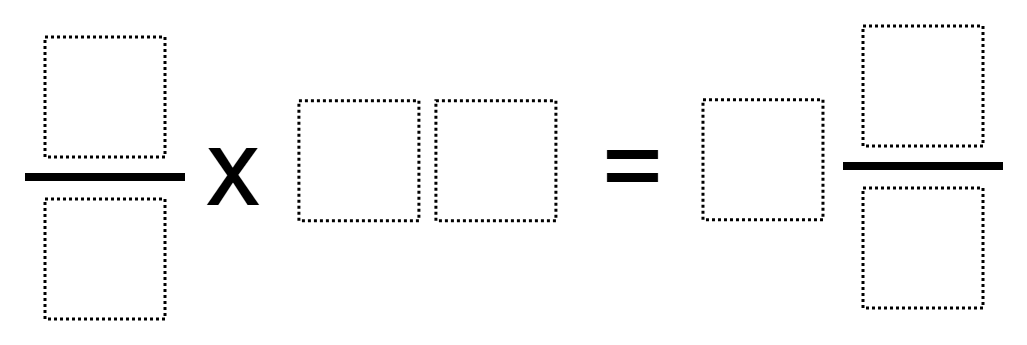# Multiplying Fraction by a 2-Digit Whole Number

Directions: Using the digits 0 through 9 at most one time, place a digit in each box to make a true equation.### Hint

How can you make sure that your product is a mixed number?
Are there some two-digit numbers that don’t divide equally into groups?
How can you rename fractions so that you don’t use the same digit more than once?

There are multiple solutions. One is 2/4 x 17 = 8 3/6.

Source: Ellen Metzger

## Add Fractions with Decimal Sums

Directions: Using the digits 1 to 9 at most one time each, place a digit …

1.Wow. This took me a while… 8 tries. But very satisfying.

2.2/4 x 15 = 7 3/6

3.4.1/3 x 08 = 2 6/9

5.If zero cannot be used to start the two-digit number:
1/4 x 23 = 5 6/8
1/8 *60=7 2/4
5/6 x10= 8 3/9
1/8 x 76 = 9 2/4
2/8 x 37=9 1/4

If zero can be used to start the two-digit number:
5/6 x 09 = 7 4/8
5/6 x 09 = 7 1/2
2/4 x 05= 7 and 3/6
3/8 x 06 = 2 1/4
4/3 x 07 = 9 2/6
1/2 x 07 = 3 4/8
5/4 x 06=7 1/2
3/4 x 07 = 5 2/8
6/8 x 07 = 5 1/4